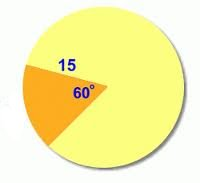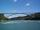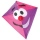# Arc

Calculate span of the arc, which is part of a circle with diameter d = 20 m and its height is 6 m.

x =  18.3303 m

### Step-by-step explanation:Did you find an error or inaccuracy? Feel free to write us. Thank you!Tips to related online calculators
Pythagorean theorem is the base for the right triangle calculator.

#### You need to know the following knowledge to solve this word math problem:

We encourage you to watch this tutorial video on this math problem:

## Related math problems and questions:

• Quarter of a circleCalculate the circumference of a quarter circle if its content is S = 314 cm2.
• BridgeThe bridge arc has a span 34 m and height 3 m. Calculate the radius of the circle arc of this bridge.
• Right isoscelesCalculate area of the isosceles right triangle which perimeter is 26 cm.
• Quarter circleWhat is the radius of a circle inscribed in the quarter circle with a radius of 100 cm?
• Height UTHow long is height in the equilateral triangle with a side b = 43?Ladder 8 m long is leaning against the wall. Its foot is 1 m away from the wall. At which height ladder touches the wall?
• Is right?Is a triangle with sides 51, 56, and 77 right triangle?Ladder 10 meters long is staying against the wall so that its bottom edge is 6 meters away from the wall. What height reaches ladder?
• Completing squareSolve the quadratic equation: m2=4m+20 using completing the square method
• Circle chordCalculate the length of the chord of the circle with radius r = 10 cm, length of which is equal to the distance from the center of the circle.
• Circular arcCalculate the length of the circular arc if the diameter d = 20cm and the angle alpha = 142 °
• CircleHow big is an area of a circle if its circumference is 51.2 cm?
• Square s3Calculate the diagonal of the square, where its area is 0.49 cm square. And also calculate its circumference.
• ChordIn a circle with radius r=60 cm is chord 4× longer than its distance from the center. What is the length of the chord?
• Triangular prismThe triangular prism has a base in the shape of a right triangle, the legs of which is 9 cm and 40 cm long. The height of the prism is 20 cm. What is its volume cm3? And the surface cm2?
• The cubeThe cube has a surface area of 486 m ^ 2. Calculate its volume.
• A kiteChildren have a kite on an 80m long rope, which floats above a place 25m from the place where children stand. How high is the dragon floating above the terrain?